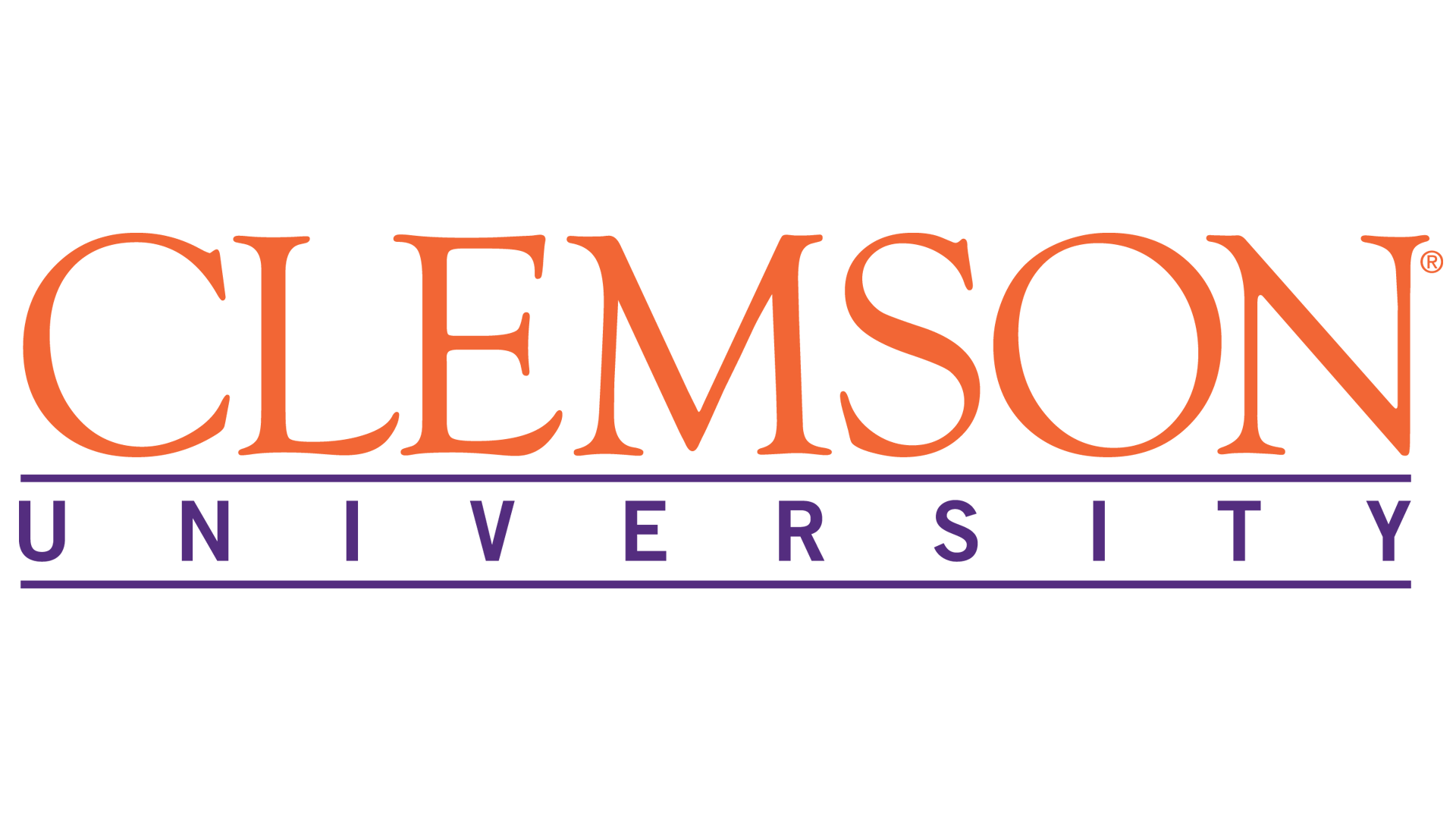# Accounting Math Professional

Increasingly, basic math – addition, subtraction, multiplication, etc. – is more and more useful in daily life. Despite the combination of letters and numbers, parentheses and exponents, algebra has just as much use as these basic functions not only in daily life, but in every job function as well. Likewise the ability to analyze and interpret data is more and more valued in this data- and information-driven world. At the root of this analysis lie the basic principles of statistics. Interpreting data for business - no matter what sector it is - is imperative to making informed decisions. And with information and data so much more widely available than ever before, there’s no excuse not to use this information to make sound business decisions every day. Algebra and statistics are fundamental skills individuals require for success regardless of the industry they enter, particularly in pursuing careers in Business or Computer Science.

This program takes students through the fundamentals of algebra and statistics as well as their endless applications. Students will learn to solve multiple types of equations including both linear and quadratic functions and master the skills necessary to solve challenging word problems, including actual scenarios one encounters on the job. Further, this program will ensure students gain the skills necessary to perform complex statistical analysis of selected samples, understand how to choose the most applicable samples for study and draw conclusions from the analysis pulled from various data sets. Students will learn to gather multiple answers to questions posed every day in business using statistical distributions, probabilities, confidence intervals and more! Once able to build applicable statistical models and interpret the data pulled together, these skills will serve students in their careers for years to come. This program presents students with the key concepts of college-level algebra and statistics. Students will be introduced to solving equations and inequalities, functions (including linear, quadratic, polynomial, rational, exponential, and logarithmic). Further, this program will offer students the skills necessary to interpret and critically evaluate statistics commonly used to describe, predict, and evaluate data in an information-driven environment.

#### Program Objectives

After completing this program, learners will be able to:

• Outline Differentiate among various processes used in manipulating algebraic expressions Analyze algebraic equations and inequalities
• Evaluate a function
• Evaluate the relationship between functions and graphs illustrating functions
• Analyze polynomial and rational functions
• Evaluate exponential and logarithmic functions
• Apply various techniques to solve systems of linear equations and inequalities
• Evaluate sequences and series
• Analyze the factorial function
• Explain the fundamentals of a statistical study
• Describe data sets and their measures in different forms
• Use descriptive statistics to describe and make statements about the data set
• Interpret probabilities outcomes
• Describe a normal distribution and make calculations using z-scores
• Use inferential statistical tests to describe population parameters
• Construct confidence intervals and use them to interpret population means
• Formulate null and research hypotheses for claims made about population means
• Use an appropriate statistical technique to test a hypothesis
• Describe the linear association for a set of paired data
• Calculate and interpret the chi-square test of independence
• Use Excel to organize data and to calculate and interpret statistical tests
• Use Microsoft Office

#### See where this program is available#### Payment Options

##### eLearningFull Payment

Pay in full and get started immediately

##### eLearning12 Payments

0% interest. 12 equal payments

#### Grant Options

No Grant options for this school yet.